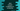# How to convert a negative number to positive in Java## Java program to convert a negative number to positive:

In this post, we will learn how to convert a negative number to positive in Java. We can solve this in two different ways. The easiest way is to multiply the number by -1. We can also use the `Math.abs` method to find the absolute value, it changes a negative value to positive.

We will write a `Java` program that will take one negative number as input from the user and print out its positive value.

### Method 1: By multiplying the number by `-1`:

In this method, we are multiplying a negative number by -1. It will give the positive value of that number. The program takes the number as input from the user and it also verifies if the number is a negative number or not.

Below is the complete program:

``````import java.util.Scanner;

public class FirstExample {

public static void main(String[] args) {
int givenNumber;
int positiveNumber;

try (Scanner sc = new Scanner(System.in)) {
System.out.println("Enter a number: ");

givenNumber = sc.nextInt();

if (givenNumber < 0) {
positiveNumber = givenNumber * (-1);
System.out.println("Positive number: " + positiveNumber);
} else {
}
}

}
}``````

• The integer variable `givenNumber` is used to assign the user-input number. The second integer variable `positiveNumber` is used to assign the converted positive value of the number.
• It uses a `Scanner` object to read the user-input value. It assigns the user input value to the `givenNumber` variable.
• If the value of `givenNumber` is negative, it multiplies that value by -1 and assigns it to the integer variable `positiveNumber` and prints that value.

If you run this program, it will print output as below:

``````Enter a number:
-23
Positive number: 23``````

### Method 2: By using `Math.abs()`:

The `abs()` is a static method defined in the `Math` class of Java. We can use this method to convert a negative number to positive. This method is defined as:

``public static int abs(int a)``

It returns the absolute value of `a`. If `a` is positive, it returns it. Else, it returns the negation value of `a`. The following program uses `Math.abs()` to convert a negative number to positive:

``````import java.util.Scanner;

public class SecondExample {

public static void main(String[] args) {
int givenNumber;
int positiveNumber;

try (Scanner sc = new Scanner(System.in)) {
System.out.println("Enter a number: ");

givenNumber = sc.nextInt();

if (givenNumber < 0) {
positiveNumber = Math.abs(givenNumber);
System.out.println("Positive number: " + positiveNumber);
}
}

}
}``````

It will give similar output.

### How to use `Math.abs` with `double`:

The above two examples uses `integer` values with the `Math.abs` method. We can also use `float`, `double` or `long` as the parameter. The `Math.abs` method is defined for all of these types:

``````public static long abs(long a){}

public static float abs(float a){}

public static double abs(double a){}``````

The following example shows how to use the `Math.abs()` method with a `double` parameter:

``````import java.util.Scanner;

public class ThirdExample {

public static void main(String[] args) {
double givenNumber;
double positiveNumber;

try (Scanner sc = new Scanner(System.in)) {
System.out.println("Enter a number: ");

givenNumber = sc.nextDouble();

if (givenNumber < 0) {
positiveNumber = Math.abs(givenNumber);
System.out.println("Positive number: " + positiveNumber);
} else {
}
}

}
}``````

``````Enter a number: